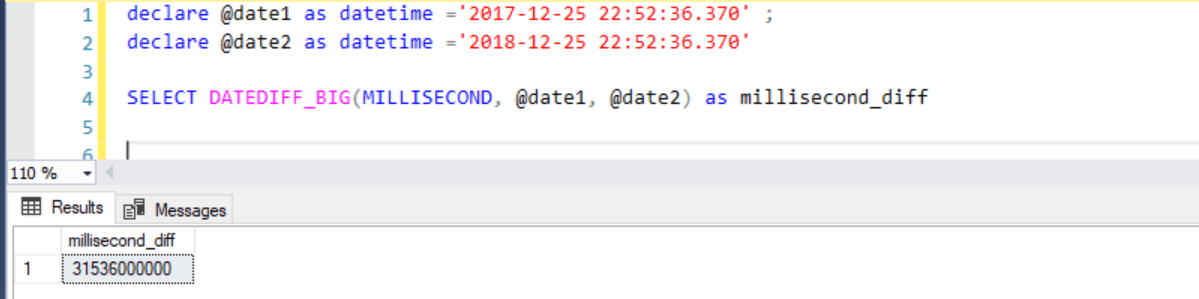Home » SQL DATEDIFF()

# SQL DATEDIFF()SQL DATEDIFF() function is used to calculate the difference between two dates.

SYNTAX

`DATEDIFF(datepart, date1, date2)`

The DATEDIFF() function accepts three arguments that is datepart, date1 and date2.

datepart is the part of date that you want to compare between date1 and date 2 and it could be a month, a year, a day etc.
date1 and date2 are the dates to be compared and it could be a type of datetime, date, time etc.

It always subtracts a date1 from date2, datepart of a date that is passed to argument 2 is always subtracted from datepart of date that is passed to argument 3  .

DATEDIFF() function returns an integer value which is the difference of datepart between date1 and date2 .

If a return value out of range for int (-2,147,483,648 to +2,147,483,647) , DATEDIFF() function returns an error , in such case you should use DATEDIFF_BIG().

Lets look at an example of DATEDIFF() in SQL Server.

#### Following SQL statement returns a differences of two dates for a datepart – month, day, year, quarter, dayofyear, week, hour and minute

```declare @date1 as datetime ='2017-12-25 22:52:36.370';
declare @date2 as datetime ='2018-01-25 22:52:36.370'

SELECT DATEDIFF(year, @date1, @date2) as year_diff,
DATEDIFF(month, @date1, @date2) as month_diff,
DATEDIFF(day, @date1, @date2) as day_diff,
DATEDIFF(quarter, @date1, @date2) as quarter_diff,
DATEDIFF(dayofyear, @date1, @date2) as dayofyear_diff,
DATEDIFF(week, @date1, @date2) as week_diff,
DATEDIFF(hour, @date1, @date2) as hour_diff,
DATEDIFF(minute, @date1, @date2) as minute_diff;```#### What if the date1 and date2 are same

If date1 and date2 are same then for any given datepart the difference will be zero (0).

```declare @date1 as datetime ='2017-12-25 22:52:36.370' ;
declare @date2 as datetime ='2017-12-25 22:52:36.370'

SELECT DATEDIFF(year, @date1, @date2) as year_diff,
DATEDIFF(month, @date1, @date2) as month_diff,
DATEDIFF(day, @date1, @date2) as day_diff,
DATEDIFF(quarter, @date1, @date2) as quarter_diff,
DATEDIFF(dayofyear, @date1, @date2) as dayofyear_diff,
DATEDIFF(week, @date1, @date2) as week_diff,
DATEDIFF(hour, @date1, @date2) as hour_diff,
DATEDIFF(minute, @date1, @date2) as minute_diff;```As you can see, for a same dates DATEDIFF() function returns 0 for any datepart.

Similarly, you can use other valid datepart in this function to get a difference between two dates.

```day                    d, dd
month                  m, mm
year                   yy, yyyy
quarter                qq, q
hour                   hh
minute                 mi, n
second                 ss, s
millisecond            ms
microsecond            mcs
nanosecond             ns
week                   wk, ww
dayofyear              dy, y```

#### what if, when the difference between two dates for the datepart milliseconds is greater than the int max (i.e. 2,147,483,647) value

In following T-SQL statement for a datepart millisecond the difference between two given dates is exceeding the max int value 2,147,483,647. In this case, it gives an error as shown below.

```declare @date1 as datetime ='2017-12-25 22:52:36.370'
declare @date2 as datetime ='2018-12-25 22:52:36.370'

SELECT DATEDIFF(MILLISECOND, @date1, @date2) as millisecond_diff```As you can see, it gives an error so to avoid an over flow error you can use DATEDIFF_BIG() function.

```declare @date1 as datetime ='2017-12-25 22:52:36.370';
declare @date2 as datetime ='2018-12-25 22:52:36.370'

SELECT DATEDIFF_BIG(MILLISECOND, @date1, @date2) as millisecond_diff```Now this time, It does not give an error and returns a difference of two date for millisecond.